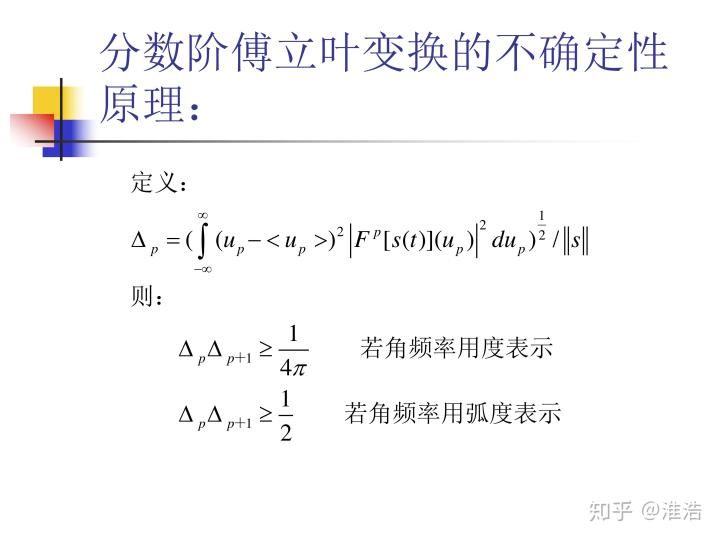• 基于高斯短时分数阶傅里叶变换的海面微动目标检测方法研究论文
• 本文研究了短时分数阶傅里叶变换(STFRFT)时频分析方法的分辨率精度和算法性能.首先,文中给出了一种STFRFT时频分辨率的数学计算表达式,其有利于时频分辨率的量化比较,仿真结果表明该理论量化值与观察值基本吻合;其次,...
• 短时分数阶傅里叶变换在尖峰海杂波中的微动目标检测与提取研究论文
• 我的分数阶傅里叶变换如下，怎么将它和短时傅里叶变换结合起来，变成短时分数阶傅里叶变换，有没有大佬指点一下下！！！frft：function Faf = frft(f, a)% The fast Fractional Fourier Transform% input: f = ...

我的分数阶傅里叶变换如下，怎么将它和短时傅里叶变换结合起来，变成短时分数阶傅里叶变换，有没有大佬指点一下下！！！

frft：

function Faf = frft(f, a)

% The fast Fractional Fourier Transform

% input: f = samples of the signal

%        a = fractional power

% output: Faf = fast Fractional Fourier transform

error(nargchk(2, 2, nargin));

f = f(:);

N = length(f);

shft = rem((0:N-1)+fix(N/2),N)+1;

sN = sqrt(N);

a = mod(a,4);

% do special cases

if (a==0), Faf = f; return; end;

if (a==2), Faf = flipud(f); return; end;

if (a==1), Faf(shft,1) = fft(f(shft))/sN; return; end

if (a==3), Faf(shft,1) = ifft(f(shft))*sN; return; end

% reduce to interval 0.5 < a < 1.5

if (a>2.0), a = a-2; f = flipud(f); end

if (a>1.5), a = a-1; f(shft,1) = fft(f(shft))/sN; end

if (a<0.5), a = a+1; f(shft,1) = ifft(f(shft))*sN; end

% the general case for 0.5 < a < 1.5

alpha = a*pi/2;

tana2 = tan(alpha/2);

sina = sin(alpha);

f = [zeros(N-1,1) ; interp(f) ; zeros(N-1,1)];

% chirp premultiplication

chrp = exp(-i*pi/N*tana2/4*(-2*N+2:2*N-2)'.^2);

f = chrp.*f;

% chirp convolution

c = pi/N/sina/4;

Faf = fconv(exp(i*c*(-(4*N-4):4*N-4)'.^2),f);

Faf = Faf(4*N-3:8*N-7)*sqrt(c/pi);

% chirp post multiplication

Faf = chrp.*Faf;

% normalizing constant

Faf = exp(-i*(1-a)*pi/4)*Faf(N:2:end-N+1);

%%%%%%%%%%%%%%%%%%%%%%%%%

function xint=interp(x)

% sinc interpolation

N = length(x);

y = zeros(2*N-1,1);

y(1:2:2*N-1) = x;

xint = fconv(y(1:2*N-1), sinc([-(2*N-3):(2*N-3)]'/2));

xint = xint(2*N-2:end-2*N+3);

%%%%%%%%%%%%%%%%%%%%%%%%%

function z = fconv(x,y)

% convolution by fft

N = length([x(:);y(:)])-1;

P = 2^nextpow2(N);

z = ifft( fft(x,P) .* fft(y,P));

z = z(1:N);

展开全文• 目前网络关于分数阶傅里叶变换可靠的代码来源主要有一下几个： （1）来源一： M.A. Kutay. fracF: Fast computation of the fractional Fourier transform, 1996. www.ee.bilkent.edu.tr/~haldun/fracF.m. （2）...
• 行业分类-电信-基于短时分数阶傅里叶变换的地震信号时频分解方法.rar
• 为了在弱光条件下由光场的强度分布求得其相位分布，利用分数阶傅里叶变换与光学系统之间的关系，基于Gerchberg-Saxton算法研究了Zernike相差的恢复问题，并进行了数值模拟。通过研究分数阶傅里叶变换与菲涅耳衍射...
• 分数阶Fourier变换是信号在时频平面内坐标轴绕原点逆时针旋转任意角度后构成的分数阶Fourier域上的表示方法，是一种广义的Fourier变换分数阶
• 根据Ozaktas1996年的文章《Digital Computation of the Fractional Fourier Transform》编写的分数阶傅里叶变换程序。
• 在雷达信处理中，分数阶傅里叶变换起着重要的作用，本文讲述其原理及实现方法
•研究论文
• 基于matlab的分数阶傅里叶变换故障诊断技术的实现
• 包含 4种 分数傅里叶变换的matlab代码实现方式，可以用于图像加密，分数傅里叶变换分析
• frft(离散傅里叶分数变换)的matlab实现代码。利用其对单分量、多分量的LFM信号进行了检测和估计，结果验证了frft的正确性。
• 压缩包中包含原图和水印图片，可以使用，只是不知道是不是参数调整有问题，效果不是太好
•研究论文
• 利用分数阶傅里叶变换对线性调频信号进行参数估计，包括中心频率和调频率，在阶次搜索的时候利用了粗搜索和精细搜索的两级搜索方法
• 针对低信噪比条件下雷达信号脉内调制方式识别算法识别率低的问题,提出了一种基于分数阶傅里叶变换(FRFT)和循环谱的雷达信号识别方法。通过分数阶傅里叶变换搜索出最大峰值对应的分数阶，把信号粗分为非调频信号和...
• 以小波变换和分数傅里叶变换为基本理论依据，介绍了一种基于小波变换分数阶傅里叶变换多图加密技术，丰富了多图加密的方法。该方法充分利用了小波变换的多分辨率分解的特性，结合分数傅里叶变换，不仅有效完成了多...
• 稀疏分数阶傅里叶变换及其在雷达运动目标检测中的应用研究论文
• 对于分数阶傅里叶变换的实现，因一般情况下分数阶傅里叶变换给不出解析表达式，故分数阶傅里叶变换的数值算法的研究是十分重要的。给出了分数阶傅里叶变换的较准确的数值计算方法。利用此方法对被线性调频函数污染...
• MATLAB工具箱大全- 分数阶傅里叶变换的程序FRFT
• 本文介绍了一种崭新的信号分析工具—分数阶...介绍了分数阶傅里叶变换的几种不同的引人途径和其基本性质之后, 在一频平面对分数阶傅里叶变换进行了研究, 用经典的傅里叶变换变换的观点对分数阶傅里叶变换进行了解释。分数阶傅里叶变换
• 离散分数阶Fourier变换(DFRFT)算法FRFT 这篇文献发表于： 作者： 一、分数阶Fourier变换的定义 二...二、分数阶傅里叶变换与Wigner-Ville分布 首先，看一下Wigner-Ville分布 是 傅里叶变换 经过一系列变换后变为 由...

离散分数阶Fourier变换(DFRFT)算法FRFT 这篇文献发表于： 作者： 一、分数阶Fourier变换的定义 二、分数阶与其他时频分析工具( Wigner-Ville分布)的关系 三、离散分数阶傅立叶变换的计算 一、分数阶Fourier变换的定义 二、分数阶傅里叶变换与Wigner-Ville分布 首先，看一下Wigner-Ville分布 是 傅里叶变换 经过一系列变换后变为 由以上可得，等式的右边是 的Wigner-Ville分布， 左边是 的Wigner-Ville分布 也就是说 的Wigner-Ville分布， 是由 的Wigner-Ville分布旋转а角得到。 所以分数阶Fourier变换有一个重要的性质，分数阶Fourier变换是角度为α的时频面旋转. 这个性质建立起分数阶Fourier变换与时频分布间的直接联系, 并且为分数阶Fourier域理解为一种统一的时频变换域奠定了理论基础, 同时也为分数阶Fourier变换在信号处理领域中的应用提供了有利条件。 t ω u v α α 三、离散分数阶傅立叶变换的计算 目前DFRFT的四种离散化算法 在这篇文献中，第二种，采用分解的方法。 1.第一种分解方法 可以把以上改写为 假定p∈[-1,1],经过量纲归一化的信号x(t)的分数阶傅里叶变换， 可以分解为以下三个步骤： (1)用chirp信号调制信号f(x): (2)调制信号与另一个chirp信号卷积: (3)用chirp信号调制卷积后的信号： 式 1 式 2 式 3 具体细节：第一步：将函数， 与线性调频函数相乘(式1)。 注意，g(x)的频率带宽与时间带宽乘积可以是，f(x)的相应带宽乘积的两倍，所以要求g(x)的采样间隔为1／(2Δx)。如果，( )样本值的采样间隔是1／Δ x，那么就需要对这些样本值进行插值，然后再与线性调频函数的离散采样值相乘，以得到所希望的g(x)的采样。 第二步：将g(x)与一线性调频函数作卷积式(式 (2))。注意，由于g(x)是带限信号，所以线性调频函数也可以用其带限形式代替而不会有任何影响。 2、第二种分解方法 为了简化计算，人们提出更加有效的分解计算方法。 假定x(t)的wigner-ville分布限定在以原点为中心，直径为Δx的 圆内。若令 ，则与chirp信号乘积后的信号 在频域具有带宽Δx。可以用Shannon插值表示 简要介绍一下Shannon 插值 Shannon定理 到设信号 ，如果存在 ，使 ， ， 则称 是B频率截断的的，这时，只要采样间隔 按间隔 进行采样就不会损失信息，而且， 可按如下公式构造原信号 上式Shannon 插值公式。 利用采样序列 3、MATLAB程序 function Faf = frft(f, a) % The fast Fractional Fourier Transform % input: f = samples of the signal % a = fractional power % output: Faf = fast Fractional Fourier transform error(nargchk(2, 2, nargin)); f = f(:); N = length(f); shft = rem((0:N-1)+fix(N/2),N)+1; sN = sqrt(N); a = mod(a,4); % do special cases if (a==0), Faf = f; return; end; if (a==2), Faf = flipud(f); return; end; if (a==1), Faf(shft,1) = fft(f(shft))/sN; return; end if (a==3), Faf(shft,1) = ifft(f(shft))*sN; return; end % reduce to interval 0.5 < a < 1.5 if (a>2.0), a = a-2; f = flipud(f); end if (a>1.5), a = a-1; f(shft,1) = fft(f(shft))/sN; end if (a<0.5), a = a+1; f(shft,1) = ifft(f(shft))*sN; end % the general case for 0.5 < a < 1.5 alpha = a*pi/2; tana2

展开全文• 鉴于人脸识别面临光照、表情和遮挡等因素的影响，提出了一种在分数阶傅里叶变换域稀疏表示的人脸识别。基于分数阶傅里叶变换对光照、表情的鲁棒性，已在图像处理领域得到应用。FRFT幅度随阶次的变换呈现压缩性，而...人脸识别 贪婪算法 特征融合
• 16 分数阶傅里叶变换的程序FRFT 17 魔方模拟器与规划求解 18 隐马尔可夫模型工具箱 HMM 19 图理论工具箱GrTheory 20 自由曲线拟合工具箱ezyfit 21 分形维数计算工具箱FracLab 2.2 22 For-Each 23 PlotPub 24 ...MATLAB 工具箱
• 对LFM信号分数阶傅里叶变换后为什么会有两个峰值看看日历，情人节到，鲜花糖果，怎能少掉，满满爱意，将你拥抱，最美是你淡淡微笑。非平稳信号广泛存在于自然界与现实生活中，作为非平稳信号的线性调频信号(Liner ...

对LFM信号分数阶傅里叶变换后为什么会有两个峰值看看日历，情人节到，鲜花糖果，怎能少掉，满满爱意，将你拥抱，最美是你淡淡微笑。

非平稳信号广泛存在于自然界与现实生活中，作为非平稳信号的线性调频信号(Liner Frequency Modulation，LFM)和正弦调频信号(Sinusoidal Frequency Modulation，SFM)广泛应用于通信、雷达、声纳、地震勘测和生物医学等众多领域。

function Faf = frft(f, a) % The fast Fractional Fourier Transform %f是信号的采样值。分享：分数阶傅里叶变换的matlab程序，请各位帮帮忙

分享：分数阶傅里叶变换的matlab程序，请各位帮帮忙 在下有礼了

function Faf = frft(f, a) % The fast Fractional Fourier Transform % input: f = samples of the signal % a = fractional power % output: Faf = fast Fractional Fourier transform error(nargchk(2, 2, nargin)); f = f(:); N = length(f)

分数阶傅里叶变换在matlab中的实现clear; smp=0.01; t=-2:smp:2; f=exp(-j*2*pi*(t.^2)); xnum=4; p=0.05;天啊，我真的那么幸运。。祝你学业有成，工作顺利，心想事成，新年快乐。。。

分数阶傅里叶变换frft数值计算，分享助

解：设f(z)=(sinz)/[z(z-1)]。当z=0、z=1时，z(z-1)=0，均位于丨z丨=2内。但由于sinz=∑[(-1)^n]z^(2n+1)/(2n+1)。，n=0,1,……，∞，∴z=0不是f(z)的极点。 ∴在丨z丨=2内，仅有1个极点z1=1，根据留数定理， ∴原式=(2πi)Resf(z1)=(2πi)lim(z→z1)(z-z1)

傅立叶变换和分数阶傅立叶变换后频率的区别 有问题就是傅立叶变换之后得出频谱 他的频率和分数阶傅立叶变换后得出的频率是变出来叫傅立十口

分数阶傅里叶变换调用前要归一化吗用MATLAB 实现傅里叶变换: 用户任意输入一个函数，然后，输出函数的傅里叶变换函数，然后输出振幅频率 。 x=sin(2*pi*t); %任意输入一个函数。 y=fft(x); %傅里叶变换函数。 plot(abs(y)); %振幅频率。

怎样利用分数阶傅立叶变换进行图像去噪，怎样写滤fft变换 lowpass滤波 ifft逆变换

比如说一条加速度-时间关系曲线，经过傅里叶变换后横坐标是频率，纵坐标跟以前代表的意义一样。

展开全文• 短时傅里叶变换 matlab源程序，供大家参考学习...